# Converting Percents To Fractions

in STEMGeeks2 years ago

Hi there. In this mathematics education guide post, I cover the topic of converting percents to fractions. The motivation behind this post was from me assisting a student in this topic for an upcoming test.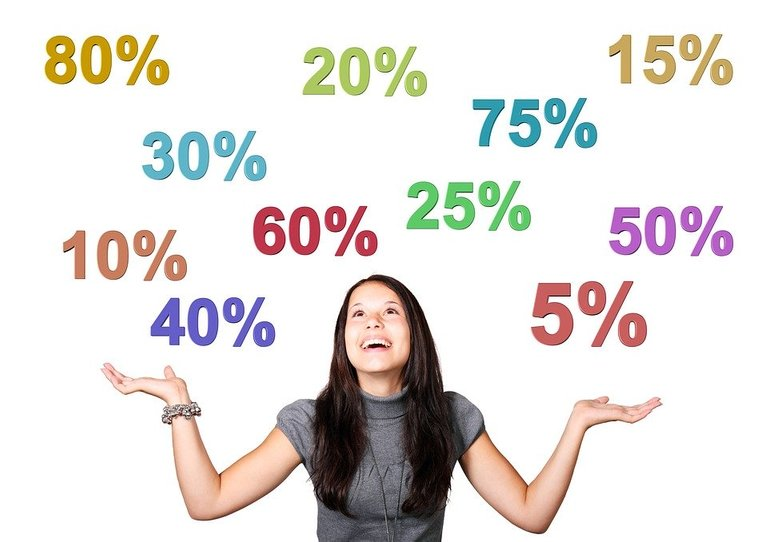Pixabay Image Source

Math text rendered in LaTeX with Quicklatex.com

## Topics

• The Meaning Of Percents
• Percents To Fractions
• Weird & Unusual Cases
• Practice Problems
• Solutions To Practice Problems

## The Meaning Of Percents

Percent refers to for each 100 or for every 100. One percent is symbolized by 1% which means 1 for every 100. Ten percent means 10 for every 100, 200% means 200 for every 100 and something like 0.5% means 0.5 for every 100.

Percents As Fractions

As a percent means one for every 100, we can convert percents into fractions. One percent would be one hundredth or 1 over 100.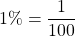Having something like 20 percent would be reduced into one fifth from 20 over 100. One fifth is in lowest terms.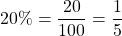As another example, three hundred percent would be `300% = 300/100 = 3`.

In general, if you have `x%` the fraction version would be: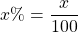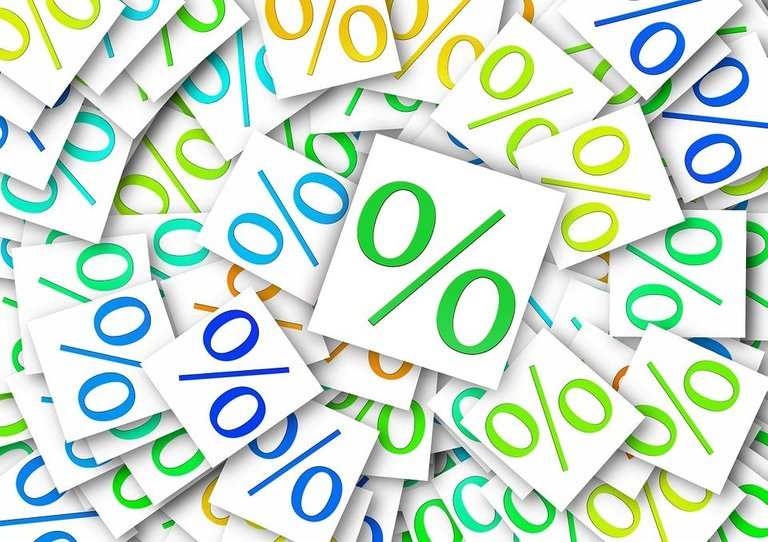Pixabay Image Source

## Fractions To Percents

The previous section had percents to fractions. What if wanted to go from fractions to percents? The main idea with fractions to percents is to convert the fraction into an equivalent fraction with a denominator (bottom number) of 100.

Example One

What is nine tenths as a percent?

Nine tenths as a fraction is 9/10. An equivalent fraction of nine tenths is 90/100 by multiplying top and bottom by 10.

Example Two

Express one quarter as a percent.

One quarter is equal to 1/4 as fraction. The 1/4 fraction needs to be converted into an equivalent fraction that has a denominator of 100. To do this, multiply the top and bottom number by 25. The corresponding percentage to one quarter is 25%.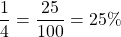Example Three

What is 3/75 as a percent?

It is not possible to convert the fraction 3/75 into an equivalent fraction with a denominator of 100 right away. However, if you reduce the 3/75 into lowest terms you obtain 1/25. The fraction 1/25 can be converted into an equivalent fraction that has 100 on the bottom.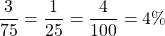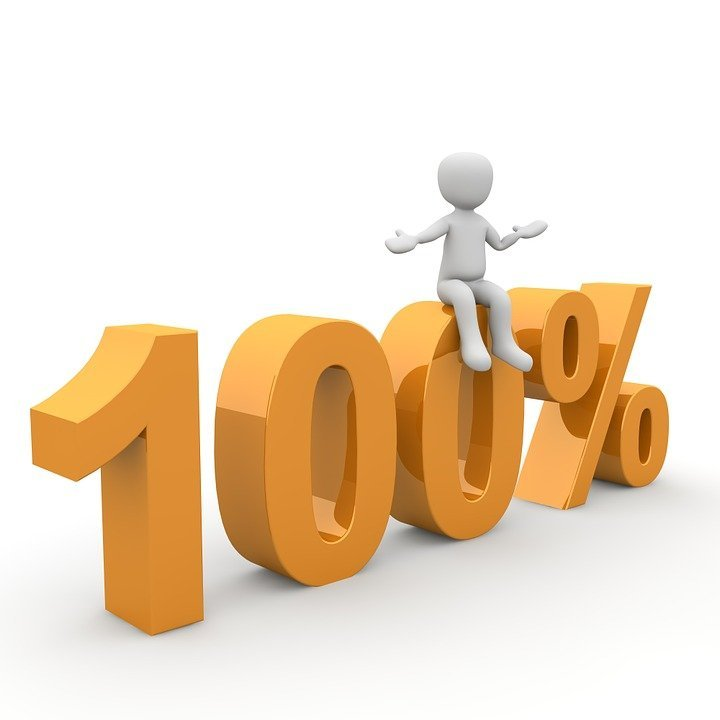Pixabay Image Source

## Weird & Unusual Cases

Decimals In Percentage Example

What is 63/1000 as a percent?

This example is different from the first two. It is because the denominator is greater than 100. Finding the equivalent fraction in this case would involve division instead of multiplication. With this example, I divide top and bottom by 10.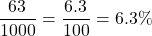Normally, decimals in a fraction are not allowed. When it comes to converting a fraction to a percentage it is okay.

Mixed Numbers To A Percentage Example 1

What is two and one tenths as a percentage?

Two and one tenths is represented as `2 1/10`. Converting this into an improper fraction would be 21/10. The equivalent fraction to 21/10 that has a denominator of 100 is 210/100.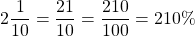Mixed Numbers To A Percentage Example 2

Convert 7 and five sevenths into a percentage.

This example is a bit more involved. Start with converting the mixed number into an improper fraction.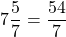As percents represent for each 100, multiply this improper fraction by 100%.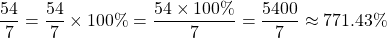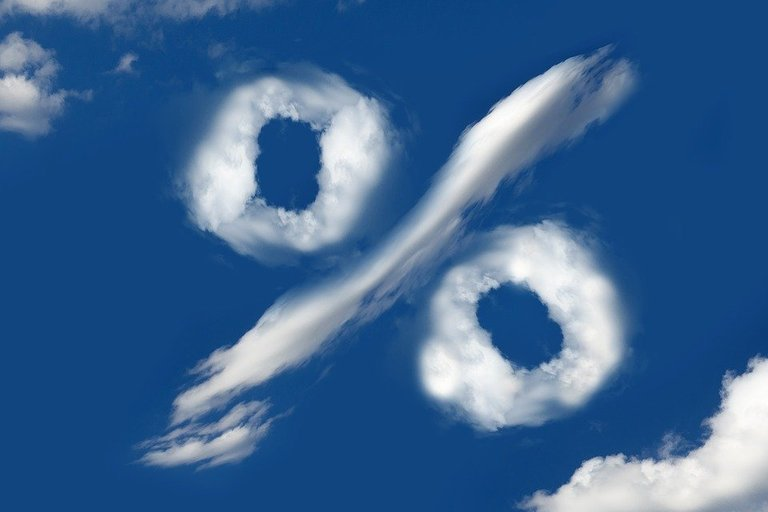Pixabay Image Source

## Practice Problems

Convert the following fractions into percents.

1. 3/4

2. 2/5

3. 11/1000

4. Three and a half

5. Eight and two ninths.

## Solutions To Practice Problems

1. 75%

2. 40%

3. 1.1%

4. 350%

5. 822.22%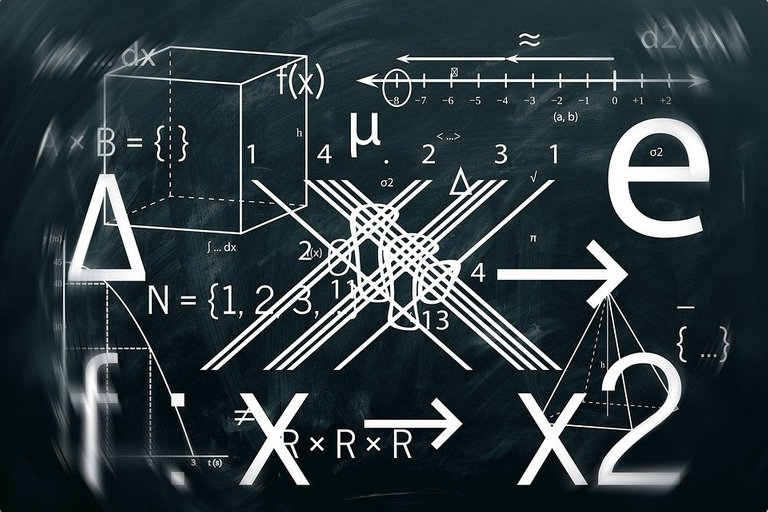Pixabay Image Source